2018-10-16 08:27:01 qq_33810188 阅读数 371
• ###### Tensorflow-图像处理视频教程

购买课程后，可扫码进入学习群，获取唐宇迪老师答疑 课程以图像处理软件Tensorflow作为核心武器，基于图像处理热点话题进行案例实战。选择当下热门模型，使用真实数据集进行实战演示，通俗讲解整个算法模型并使用tensorflow进行实战，详解其中的原理与代码实现。

1313 人正在学习 去看看 唐宇迪

· 能从硬件结构方面消除不利影响，最好不要从图像算法方面消除不利影响（预防隐患法则）

· 截至目前为止，图像算法没有一个标准的算法流程适合所有的图像处理，所以具体问题具体分析

· 图像算法处理的实际应用遵循海森堡不确定性原则，所以在实际应用中不断优化算法十分必要

· 图像算法处理的实际应用符合墨菲定律，所以图像算法前期的设计隐患一定会在实际应用中产生

· 图像处理算法一定是定量分析算法，模糊不定的处理方式必然会导致问题

· 图像处理问题归根结底是一个数学问题，所以扎实的数学功底必不可少！

---------------------------------------------------------------------------------------------------------------

1.图像算法的设计一定要考虑数据结构类型，不友好的数据结构会使后续的代码结构十分繁琐冗余！

2.无论函数名，还是参数名，在取名的时候千万避免名称相似（例如，如果有一个camsPixels，不可再取一个camPixels，否则在查看代码时，直观上很容易分辨错误！浪费解读代码时间！）；

3.不要重复命名函数，否则哪天封装多个带有重复名称的函数时，会出现命名冲突！

4.尽量不要重复造轮子，能够借鉴的代码要借鉴并优化，不断学习别人的代码风格和代码逻辑；

5.一位优秀的算法工程师，也必须是一位不错的软件工程师！

6.

2017-11-24 22:42:45 xxxqcbQ 阅读数 255
• ###### Tensorflow-图像处理视频教程

购买课程后，可扫码进入学习群，获取唐宇迪老师答疑 课程以图像处理软件Tensorflow作为核心武器，基于图像处理热点话题进行案例实战。选择当下热门模型，使用真实数据集进行实战演示，通俗讲解整个算法模型并使用tensorflow进行实战，详解其中的原理与代码实现。

1313 人正在学习 去看看 唐宇迪

一直想记录自己图像处理算法修炼心路历程，今天终于鼓足勇气写下第一篇，万事开头难。

算法写了快4个月了，目前写的比较多的是基础算法，比如通用的圆、直线检测算法，还真不能小瞧这类算法，想在工程上做到又快又准，真的不是一件容易的事情。这里应该算是有bug的，又快又好应当有个明确的量化指标。

对于圆检测的基本逻辑是：Sobel/Canny算子处理→拿出边界→边界筛选→最小二乘拟合(对噪声很敏感)。

2014-06-07 10:46:19 zhuangxiaobin 阅读数 2443
• ###### Tensorflow-图像处理视频教程

购买课程后，可扫码进入学习群，获取唐宇迪老师答疑 课程以图像处理软件Tensorflow作为核心武器，基于图像处理热点话题进行案例实战。选择当下热门模型，使用真实数据集进行实战演示，通俗讲解整个算法模型并使用tensorflow进行实战，详解其中的原理与代码实现。

1313 人正在学习 去看看 唐宇迪

Scriptol列出了几种神奇的图像处理算法，让我们一起来看一下。2018-08-26 14:27:40 fanyun_01 阅读数 5170
• ###### Tensorflow-图像处理视频教程

购买课程后，可扫码进入学习群，获取唐宇迪老师答疑 课程以图像处理软件Tensorflow作为核心武器，基于图像处理热点话题进行案例实战。选择当下热门模型，使用真实数据集进行实战演示，通俗讲解整个算法模型并使用tensorflow进行实战，详解其中的原理与代码实现。

1313 人正在学习 去看看 唐宇迪

``````//添加椒盐噪声
void salt(Mat& src,int number)
{
for (int i = 0; i < number; i++)
{
int r = static_cast<int>(rng.uniform(0, src.rows));
int c = static_cast<int>(rng.uniform(0, src.cols));
int k = (static_cast<int>(rng.uniform(0, 1000))&1);
if(k==1)
src.at<uchar>(r, c) = 255;
else
src.at<uchar>(r, c) = 0;
}
return;
}
``````
``````/*
* @ drt ：高斯方差
* @ Medium ：高斯均值
*/
int Get_Gauss(int Medium, int drt)
{
//产生高斯样本，以U为均值，D为均方差
double sum = 0;
for (int i = 0; i<12; i++) sum += rand() / 32767.00;
//计算机中rand()函数为－32767～＋32767（2^15-1）
//故sum＋为0～1之间的均匀随机变量
return int(Medium + drt*(sum - 6));
//产生均值为U，标准差为D的高斯分布的样本，并返回
}
/*
* variance ：高斯噪声的方差
*/

//添加高斯噪声
void ImgAddGaussNoise1(const uchar *srcimgbuff, uchar * dstImgbuff, int srcwith, int srcheigh, int chanels)
{
assert(srcimgbuff != NULL && srcwith > 0 && srcheigh > 0);
int bytecount = srcwith * srcheigh * chanels;

for (size_t i = 0; i < bytecount; i++)
{
dstImgbuff[i] += Get_Gauss(20, 0.02);
}
}
``````
``````//中值求取
void Media(Mat* src, int indexrows, int indexcols, int* meanv, int ker)
{
int lo = (ker - 1) / 2;
vector<int>moreo;
for (int i = indexrows - lo; i <= indexrows + lo; i++)
{
for (int j = indexcols - lo; j <= indexcols + lo; j++)
{
moreo.push_back(src->at<uchar>(i, j));
}
}
sort(moreo.begin(), moreo.end());
*meanv = moreo.at(ker * ker / 2);
return;
}
``````
``````//均值求取
void Meanvalue(Mat* src, int indexrows, int indexcols, float* meanv, int ker)
{
int lo = (ker - 1) / 2;
float total = 0;
for (int i = indexrows - lo; i <= indexrows + lo; i++)
{
for (int j = indexcols - lo; j <= indexcols + lo; j++)
{
total += src->at<uchar>(i, j);
}
}
*meanv = total / (ker * ker);
return;
}
``````
``````//像素方差
void Variance(Mat& src, vector<test>& hierachy, int ker)
{
int row = src.rows;
int col = src.cols;
int lo = (ker - 1) / 2;
for (int ir = lo; ir < row - lo; ir++)
{
for (int jc = lo; jc < col - lo; jc++)
{
float means;
int var;
//计算均值
Meanvalue(&src, ir, jc, &means, ker);
Vvalue(&src, ir, jc, &var, ker, means);
test temp;
temp.menval = var;
temp.x = ir;
temp.y = jc;
hierachy.push_back(temp);
}
}
return;
}
``````
``````//局部方差求取
void Vvalue(Mat* src, int indexrows, int indexcols, int* vall, int ker, float mean)
{
int lo = (ker - 1) / 2;
float total = 0;
for (int i = indexrows - lo; i <= indexrows + lo; i++)
{
for (int j = indexcols - lo; j <= indexcols + lo; j++)
{
total += pow((src->at<uchar>(i, j) - mean), 2);
}
}
*vall = static_cast<int>(total);
return;
}
``````
``````//STL排序方式
bool SortByM1(const test &v1, const test &v2)//注意：本函数的参数的类型一定要与vector中元素的类型一致
{
return v1.menval < v2.menval;//升序排列
}
``````
``````//SSIM 结构相似比
Scalar getMSSIM(const Mat& i1, const Mat& i2)
{
const double C1 = 6.5025, C2 = 58.5225;
/***************************** INITS **********************************/
int d = CV_32F;

Mat I1, I2;
i1.convertTo(I1, d);           // cannot calculate on one byte large values
i2.convertTo(I2, d);

int num = I1.channels();
//cv::imshow("123", I1);
//cv::waitKey();

Mat I2_2 = I2.mul(I2);        // I2^2
Mat I1_2 = I1.mul(I1);        // I1^2
Mat I1_I2 = I1.mul(I2);        // I1 * I2

/*************************** END INITS **********************************/

Mat mu1, mu2;   // PRELIMINARY COMPUTING
GaussianBlur(I1, mu1, Size(11, 11), 1.5);
GaussianBlur(I2, mu2, Size(11, 11), 1.5);

Mat mu1_2 = mu1.mul(mu1);
Mat mu2_2 = mu2.mul(mu2);
Mat mu1_mu2 = mu1.mul(mu2);

Mat sigma1_2, sigma2_2, sigma12;

GaussianBlur(I1_2, sigma1_2, Size(11, 11), 1.5);
sigma1_2 -= mu1_2;

GaussianBlur(I2_2, sigma2_2, Size(11, 11), 1.5);
sigma2_2 -= mu2_2;

GaussianBlur(I1_I2, sigma12, Size(11, 11), 1.5);
sigma12 -= mu1_mu2;

///////////////////////////////// FORMULA ////////////////////////////////
Mat t1, t2, t3;

t1 = 2 * mu1_mu2 + C1;
t2 = 2 * sigma12 + C2;
t3 = t1.mul(t2);              // t3 = ((2*mu1_mu2 + C1).*(2*sigma12 + C2))

t1 = mu1_2 + mu2_2 + C1;
t2 = sigma1_2 + sigma2_2 + C2;
t1 = t1.mul(t2);               // t1 =((mu1_2 + mu2_2 + C1).*(sigma1_2 + sigma2_2 + C2))

Mat ssim_map;
divide(t3, t1, ssim_map);      // ssim_map =  t3./t1;

Scalar mssim = mean(ssim_map); // mssim = average of ssim map
return mssim;
}
``````

2016-07-03 21:03:51 qq_26499769 阅读数 10188
• ###### Tensorflow-图像处理视频教程

购买课程后，可扫码进入学习群，获取唐宇迪老师答疑 课程以图像处理软件Tensorflow作为核心武器，基于图像处理热点话题进行案例实战。选择当下热门模型，使用真实数据集进行实战演示，通俗讲解整个算法模型并使用tensorflow进行实战，详解其中的原理与代码实现。

1313 人正在学习 去看看 唐宇迪

1、图像镶嵌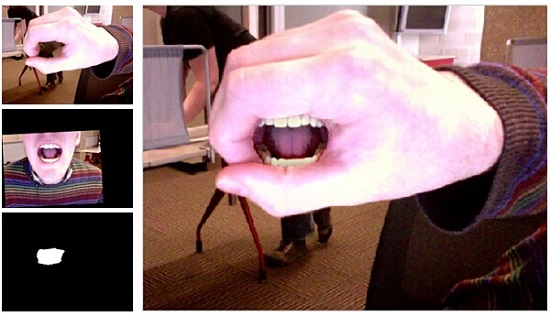2、黑白图像着色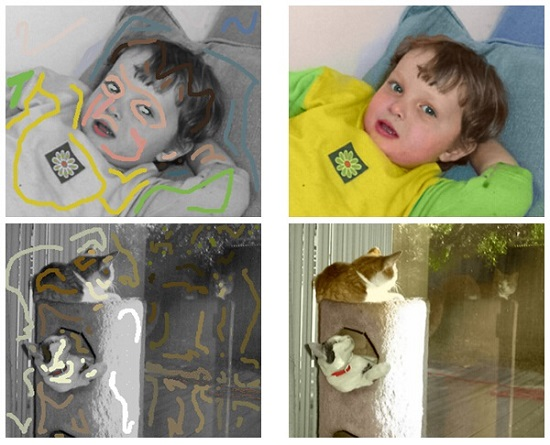3、图像超分辨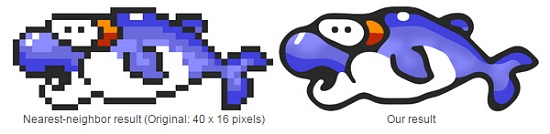4、图像去模糊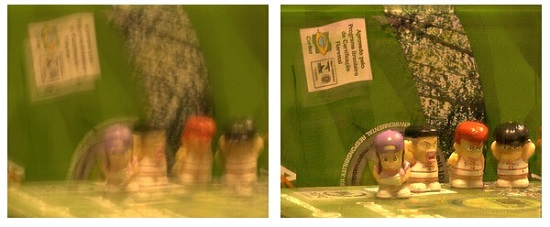5、图像编辑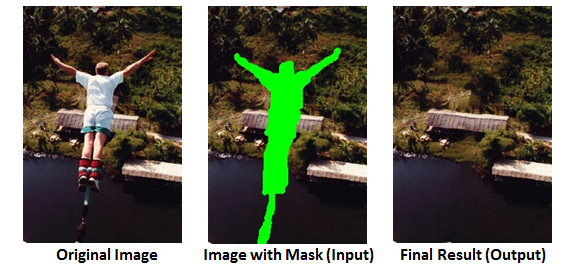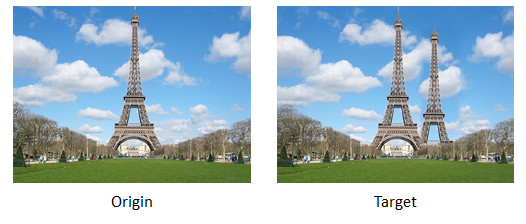Peter J. Burt and Edward H. Adelson. 1983. A multiresolution spline with application to image mosaics. ACM Trans. Graph. 2, 4 (October 1983)

 Patrick Pérez, Michel Gangnet, and Andrew Blake. 2003. Poisson image editing. ACM Trans. Graph. 22, 3 (July 2003)

 Anat Levin, Dani Lischinski, and Yair Weiss. 2004. Colorization using optimization. ACM Trans. Graph. 23, 3 (August 2004)

 Johannes Kopf and Dani Lischinski. 2011. Depixelizing Pixel ArtACM Transactions on Graphics (Proceedings of SIGGRAPH 2011).

 Xu, Li, and Jiaya Jia. 2010. Two-phase kernel estimation for robust motion deblurring.ECCV. Springer Berlin Heidelberg, 2010. 157-170.

 Y. Wexler, E. Shechtman and M. Irani "Space-Time Video Completion" Computer Vision and Pattern Recognition (CVPR), Washington, June 2004.

 Connelly Barnes, Eli Shechtman, Adam Finkelstein, and Dan B Goldman. 2009.PatchMatch: a randomized correspondence algorithm for structural image editing. ACM Trans. Graph. 28, 3, Article 24 (July 2009)

 Connelly Barnes, Eli Shechtman, Dan B Goldman, The Generalized PatchMatch Correspondence Algorithm, ECCV, 2010Depixelizing（http://research.microsoft.com/en-us/um/people/kopf/pixelart/）算法可以让低分辨率的像素图转化为高质量的向量图。